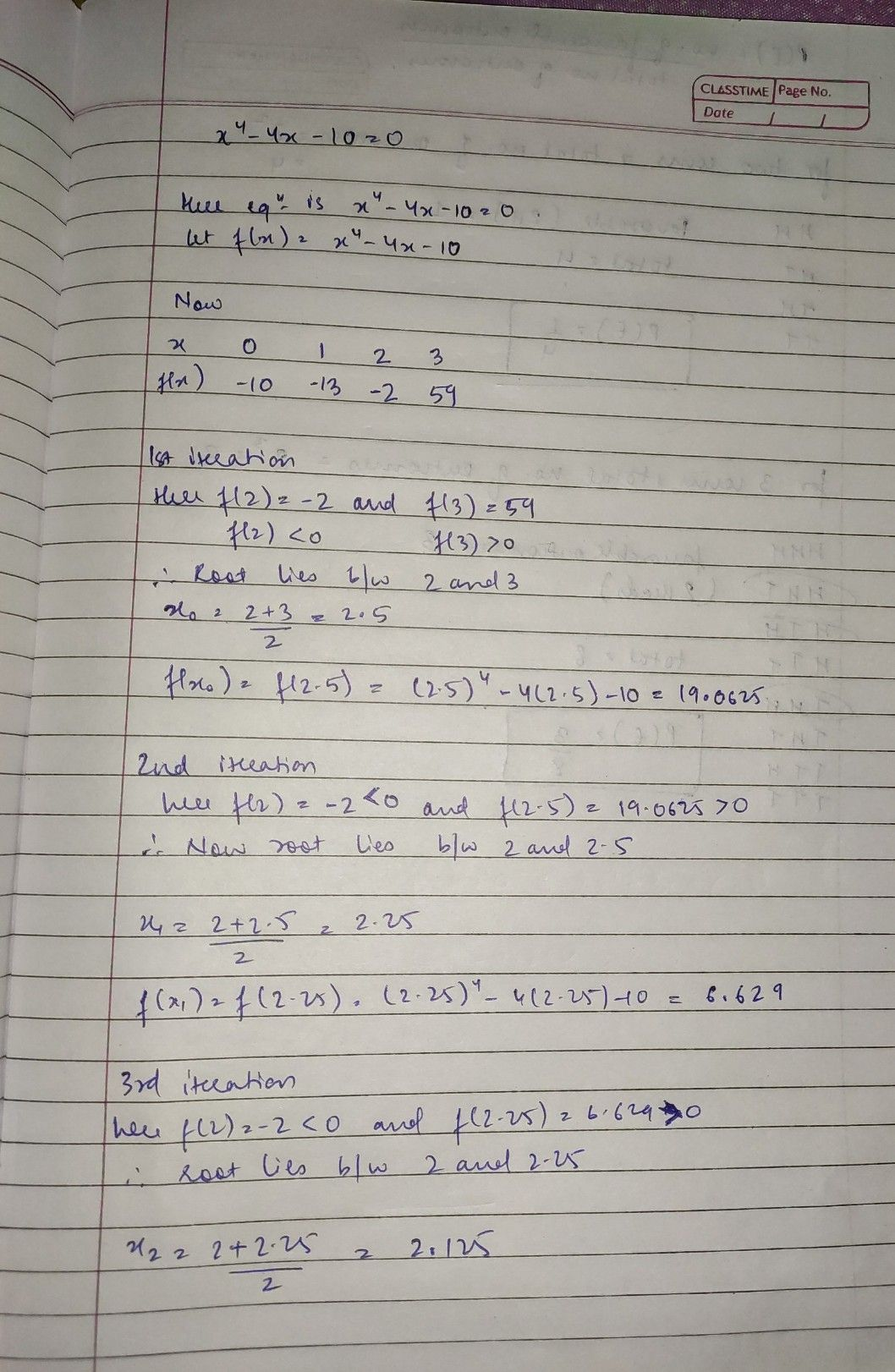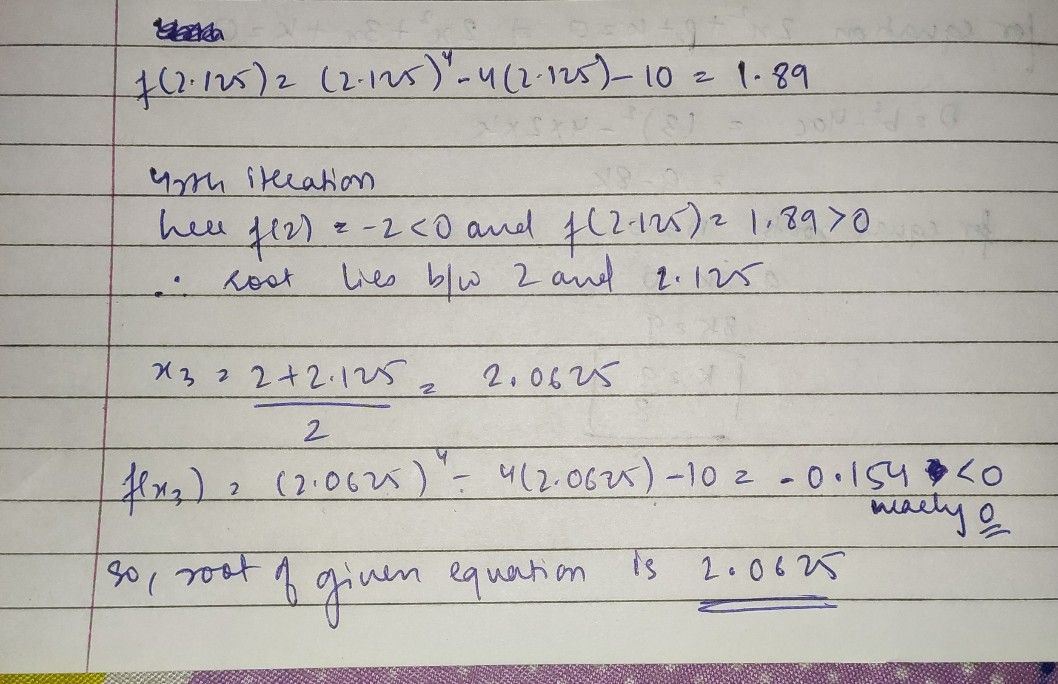Symbol
Problemmple $90$ Find a real root of the equation $x^{4}-4x-10=0$ using bisection method. $111$ (H.P.U. Ap The given equation is $x^{4}-4x-10=0$ $\right)$ $a$ $14$
Other
Search count: 107
SolutionQanda teacher - gaurav sir